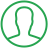# How To Plot Histogram In Python

Last Updated : Oct 16, 2023In this article we will show you the solution of how to plot histogram in python, a histogram primarily serves as a means of displaying data presented in the form of several groups.It is an accurate way for displaying the distribution of numerical data graphically.

It is a type of bar plot where the X-axis shows the bin ranges and the Y-axis provides frequency information.

The first step in creating a histogram is to establish a bin for each range of values.

Next, divide the entire range of values into a series of intervals, and then count the values that fall into each interval.

Bins are distinguished as a series of non-overlapping, successive intervals of variables.

To calculate and produce the histogram of x, use the matplotlib.pyplot.hist() function. We will now discuss the idea of how to plot histogram in python with an example.

## Step By Step Guide On How To Plot Histogram In Python :-

```import matplotlib.pyplot as plt
import numpy as np
from matplotlib import colors
from matplotlib.ticker import PercentFormatter
np.random.seed(23685752)
N_points = 10000
n_bins = 20
x = np.random.randn(N_points)
y = .8 ** x + np.random.randn(10000) + 25
legend = ['distribution']
fig, axs = plt.subplots(1, 1,
figsize =(10, 7),
tight_layout = True)
for s in ['top', 'bottom', 'left', 'right']:
axs.spines[s].set_visible(False)
axs.xaxis.set_ticks_position('none')
axs.yaxis.set_ticks_position('none')
axs.grid(b = True, color ='grey',
linestyle ='-.', linewidth = 0.5,
alpha = 0.6)
fig.text(0.9, 0.15, 'AmrutaShahane25',
fontsize = 12,
color ='red',
ha ='right',
va ='bottom',
alpha = 0.7)
N, bins, patches = axs.hist(x, bins = n_bins)
fracs = ((N**(1 / 5)) / N.max())
norm = colors.Normalize(fracs.min(), fracs.max())
for thisfrac, thispatch in zip(fracs, patches):
color = plt.cm.viridis(norm(thisfrac))
thispatch.set_facecolor(color)
plt.xlabel("X-axis")
plt.ylabel("y-axis")
plt.legend(legend)
plt.title('Customized histogram')
plt.show()```
1. import the required libraries.
2. Set the random seed to make sure the random data can be repeated.
3. Define the histogram's N_points and n_bins values for the number of data points and bins, respectively.
4. Create the x-axis (x) and y-axis (y) random data. Here, we add some random noise and utilize a power function for y and a normal distribution for x.
5. Create a legend that will be displayed in the plot for the distribution.
6. Utilize plt.subplots() to create an object with a figure and axes. Set the figure's size and allow for a compact arrangement of the elements.
7. Remove the axes' boundaries, or spines.
8. Ticks on the x-axis and y-axis should be removed.
10. Grid the plot with lines.
11. Put a text watermark in the bottom right corner of the figure.
12. Utilize axs.hist() to produce the histogram. Keep bin edges in bins and histogram counts in N.
13. The normalized bin counts should be used to determine the color of the histogram's bars.
14. Include extra elements in the plot, such as a legend, title, and labels for the x- and y-axes.
15. Plot can be displayed using plt.show().

## Conclusion :-

As a result, we have successfully learned how to plot histogram in python with an example.

As a result, we wrap up two crucial topics with charting in this Python Histogram, histograms and bar charts.

Though they appear identical, they are actually two distinct objects. Additionally, we talked about Python bar plotting examples and histogram examples.

I hope this article on how to plot histogram in python helps you and the steps and method mentioned above are easy to follow and implement.

##About Dikshita

Passionate Electronics and Communication Engineering student with expertise in web development (HTML, CSS, JS,PHP, Bootstrap, React.js) and content writing. Eager problem solver and tech enthusiast, adept at creating engaging web experiences.